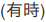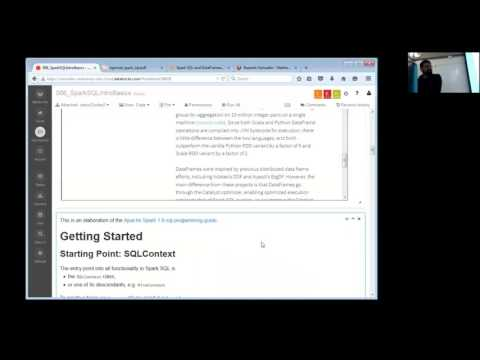// Databricks notebook source exported at Sat, 18 Jun 2016 08:36:28 UTC

# Scalable Data Science

### prepared by Raazesh Sainudiin and Sivanand Sivaram

The html source url of this databricks notebook and its recorded Uji:### Diamonds ML Pipeline Workflow - DataFrame ETL and EDA Part

This is the Spark SQL parts that are focussed on extract-transform-Load (ETL) and exploratory-data-analysis (EDA) parts of an end-to-end example of a Machine Learning (ML) workflow.

This is a scalarific break-down of the pythonic Diamonds ML Pipeline Workflow in Databricks Guide.

We will see this example gain in the sequel

For this example, we analyze the Diamonds dataset from the R Datasets hosted on DBC.

Later on, we will use the DecisionTree algorithm to predict the price of a diamond from its characteristics.

Here is an outline of our pipeline:

• Step 2. Understand the data: Compute statistics and create visualizations to get a better understanding of the data.
• Step 3. Hold out data: Split the data randomly into training and test sets. We will not look at the test data until after learning.
• Step 4. On the training dataset:
• Extract features: We will index categorical (String-valued) features so that DecisionTree can handle them.
• Learn a model: Run DecisionTree to learn how to predict a diamond’s price from a description of the diamond.
• Tune the model: Tune the tree depth (complexity) using the training data. (This process is also called model selection.)
• Step 5. Evaluate the model: Now look at the test dataset. Compare the initial model with the tuned model to see the benefit of tuning parameters.
• Step 6. Understand the model: We will examine the learned model and results to gain further insight.

In this notebook, we will only cover Step 1 and Step 2. above. The other Steps will be revisited in the sequel.

### Step 1. Load data as DataFrame

This section loads a dataset as a DataFrame and examines a few rows of it to understand the schema.

``````
// We'll use the Diamonds dataset from the R datasets hosted on DBC.
val diamondsFilePath = "dbfs:/databricks-datasets/Rdatasets/data-001/csv/ggplot2/diamonds.csv"

``````
``````
sc.textFile(diamondsFilePath).take(2) // looks like a csv file as it should

``````
``````
.format("com.databricks.spark.csv") // use spark.csv package
.option("inferSchema", "true") // Automatically infer data types
//.option("delimiter", ",") // Specify the delimiter as comma or ',' DEFAULT

``````
``````
//There are 10 columns.  We will try to predict the price of diamonds, treating the other 9 columns as features.
diamondsRawDF.printSchema()

``````

Note: `(nullable = true)` simply means if the value is allowed to be `null`.

Let us count the number of rows in `diamondsDF`.

``````
diamondsRawDF.count() // Ctrl+Enter

``````

So there are 53940 records or rows in the DataFrame.

Use the `show(n)` method to see the first `n` (default is 20) rows of the DataFrame, as folows:

``````
diamondsRawDF.show()

``````

If you notice the schema of `diamondsRawDF` you will see that the automatic schema inference of `SqlContext.read` method has cast the values in the column `price` as `integer`.

To cleanup:

• let’s recast the column `price` as `double` for downstream ML tasks later and
• let’s also get rid of the first column of row indices.
``````
import org.apache.spark.sql.types.DoubleType
//we will convert price column from int to double later
val diamondsDF = diamondsRawDF.select(\$"carat", \$"cut", \$"color", \$"clarity", \$"depth", \$"table",\$"price".cast(DoubleType).as("price"), \$"x", \$"y", \$"z")
diamondsDF.cache() // let's cache it for reuse
diamondsDF.printSchema // print schema

``````
``````
//View DataFrame
display(diamondsDF)

``````

### Step 2. Understand the data

Let’s examine the data to get a better understanding of what is there. We only examine a couple of features (columns), but it gives an idea of the type of exploration you might do to understand a new dataset.

For more examples of using Databricks’s visualization to explore a dataset, see the Visualizations Notebook.

We can see that we have a mix of

• categorical features (`cut`, `color`, `clarity`) and
• continuous features (`depth`, `x`, `y`, `z`).
##### Let’s first look at the categorical features.

You can also select one or more individual columns using so-called DataFrame API.

Let us `select` the column `cut` from `diamondsDF` and create a new DataFrame called `cutsDF` and then display it as follows:

``````
val cutsDF = diamondsDF.select("cut") // Shift+Enter

``````
``````
display(cutsDF) // Ctrl+Enter

``````

Let us use `distinct` to find the distinct types of `cut`s in the dataset.

``````
// View distinct diamond cuts in dataset
val cutsDistinctDF = diamondsDF.select("cut").distinct()

``````
``````
cutsDistinctDF.show()

``````

Clearly, there are just 5 kinds of cuts.

``````
// View distinct diamond colors in dataset
val colorsDistinctDF = diamondsDF.select("color").distinct() //.collect()
colorsDistinctDF.show()

``````
``````
// View distinct diamond clarities in dataset
val claritiesDistinctDF = diamondsDF.select("clarity").distinct() // .collect()
claritiesDistinctDF.show()

``````
``````
display(claritiesDistinctDF) // can use databricks' "magical visualizer"

``````

We can examine the distribution of a particular feature by using display(),

You Try!

1. Click on the chart icon and Plot Options, and setting:
• Value=`<id>`
• Series groupings=’cut’
• and Aggregation=`COUNT`.
2. You can also try this using columns “color” and “clarity”
``````
display(diamondsDF.select("cut"))

``````
``````
// come on do the same for color NOW!

``````
``````
// and clarity too...

``````

Now let’s examine one of the continuous features as an example.

``````
//Select: "Plot Options..." --> "Display type" --> "histogram plot" and choose to "Plot over all results"
display(diamondsDF.select("carat"))

``````

The above histogram of the diamonds’ carat ratings shows that carats have a skewed distribution: Many diamonds are small, but there are a number of diamonds in the dataset which are much larger.

• Extremely skewed distributions can cause problems for some algorithms (e.g., Linear Regression).
• However, Decision Trees handle skewed distributions very naturally.

Note: When you call `display` to create a histogram like that above, it will plot using a subsample from the dataset (for efficiency), but you can plot using the full dataset by selecting “Plot over all results”. For our dataset, the two plots can actually look very different due to the long-tailed distribution.

We will not examine the label distribution for now. It can be helpful to examine the label distribution, but it is best to do so only on the training set, not on the test set which we will hold out for evaluation. These will be seen in the sequel

You Try! Of course knock youself out visually exploring the dataset more…

``````
display(diamondsDF.select("cut","carat"))

``````

Try scatter plot to see pairwise scatter plots of continuous features.

``````
display(diamondsDF) //Ctrl+Enter

``````

Note that columns of type string are not in the scatter plot!

``````
diamondsDF.printSchema // Ctrl+Enter

``````

### Let us run through some basic inteactive SQL queries next

• HiveQL supports =, <, >, <=, >= and != operators. It also supports LIKE operator for fuzzy matching of Strings
• Enclose Strings in single quotes
• Multiple conditions can be combined using `and` and `or`
• Enclose conditions in `()` for precedence

Why do I need to learn interactive SQL queries?

Such queries in the widely known declarative SQL language can help us explore the data and thereby inform the modeling process!!!

Using DataFrame API, we can apply a `filter` after `select` to transform the DataFrame `diamondsDF` to the new DataFrame `diamondsDColoredDF`.

Below, `\$` is an alias for column.

Let as select the columns named `carat`, `colour`, `price` where `color` value is equal to `D`.

``````
val diamondsDColoredDF = diamondsDF.select(\$"carat", \$"color", \$"price").filter(\$"color" === "D") // Shift+Enter

``````
``````
display(diamondsDColoredDF) // Ctrl+Enter

``````

As you can see all the colors are now ‘D’.

Let’s try to do the same in SQL for those who know SQL from before.

First we need to see if the table is registerd (not just the DataFrame), and if not we ened to register our DataFrame as a temporary table.

``````
sqlContext.tables.show() // Ctrl+Enter to see available tables

``````

Looks like diamonds is already there (if not just execute the following cell).

``````
// uncomment next line and Ctrl+Enter to remove any temporary table named diamonds ONLY_IF it already exists!
//sqlContext.dropTempTable("diamonds")

``````
``````
// Shift+Enter to make a temporary table named diamonds from the diamondsDF
diamondsDF.registerTempTable("diamonds") // it will re-register new table if another temp table named diamonds already exist

``````
``````
%sql -- Shift+Enter to do the same in SQL
select carat, color, price from diamonds where color='D'

``````

Alternatively, one could just write the SQL statement in scala to create a new DataFrame `diamondsDColoredDF_FromTable` from the table `diamonds` and display it, as follows:

``````
val diamondsDColoredDF_FromTable = sqlContext.sql("select carat, color, price from diamonds where color='D'") // Shift+Enter

``````
``````
display(diamondsDColoredDF_FromTable) // Ctrl+Enter to see the same DF!

``````
``````
// You can also use the familiar wildchard character '%' when matching Strings
display(sqlContext.sql("select * from diamonds where clarity LIKE 'V%'"))

``````
``````
// Combining conditions
display(sqlContext.sql("select * from diamonds where clarity LIKE 'V%' and price > 10000"))

``````
``````
// selecting a subset of fields
display(sqlContext.sql("select carat, clarity, price from diamonds where color = 'D'"))

``````
``````
//renaming a field using as
display(sqlContext.sql("select carat as carrot, clarity, price from diamonds"))

``````
``````
//sorting
display(sqlContext.sql("select carat, clarity, price from diamonds order by price desc"))

``````
``````
diamondsDF.printSchema // since prince is integer in the DF turned into table we can rely on the descenting sort on integers

``````
``````
// sort by multiple fields
display(sqlContext.sql("select carat, clarity, price from diamonds order by carat asc, price desc"))

``````
``````
// use this to type cast strings into Int when the table is loaded with string-valued columns
//display(sqlContext.sql("select cast(carat as Int) as carat, clarity, cast(price as Int) as price from diamond order by carat asc, price desc"))

``````
``````
// sort by multiple fields and limit to first 5
display(sqlContext.sql("select carat, clarity, price from diamonds order by carat desc, price desc limit 5"))

``````
``````
//aggregate functions
display(sqlContext.sql("select avg(price) as avgprice from diamonds"))

``````
``````
//average operator seems to be doing an auto-type conversion from int to double
display(sqlContext.sql("select avg(cast(price as Double)) as avgprice from diamonds"))

``````
``````
//aggregate function and grouping
display(sqlContext.sql("select color, avg(price) as avgprice from diamonds group by color"))

``````

### Why do we need to know these interactive SQL queries?

Such queries can help us explore the data and thereby inform the modeling process!!!

Of course, if you don’t know SQL then don’t worry, we will be doing these things in scala using DataFrames.

Finally, those who are planning to take the Spark Develope Exams online, then you can’t escape from SQL questions there…

#### Further Preparation

For more on SQL syntax, check the SQL tutorial on W3Schools
Note that HiveQL supports only a subset of operations supported by SQL

Updated: